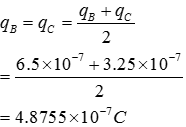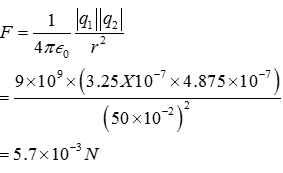# Topic: Electric Charges and Fields (Test 1)

Topic: Electric Charges and Fields
Q.1
An oil drop of 12 excess electrons is held stationary under a constant electric field of in Millikan’s oil drop experiment. The density of the oil is 1.26 g cm-3. Estimate the radius of the drop. (g = 9.81 m ; e = 1.60 × 10-19 C).
A. mm
B. mm
C. mm
D. mm
Explaination / Solution:

Density of oil, ρ = 1.26 gm/ = 1.26 ×  kg/ Acceleration due to gravity, g = 9.81 m Charge on an electron, e = 1.6 ×  C Radius of the oil drop = r Force (F) due to electric field E is equal to the weight of the oil drop (W) F = W Eq = mg Ene=mg Where,q = Net charge on the oil drop = ne m = Mass of the oil drop= Volume of the oil drop × Density of oil So 2.55×(12×1.6×)= 1.26X × So r = 9.82 ×  mm Therefore, the radius of the oil drop is 9.82 × mm.

Workspace
Report
Q.2
Which of the following is not true for a region with uniform electric field?
A. it can have free charges
B. none of these
C. it may have uniformly distributed charge
D. it may contain dipoles
Explaination / Solution:

In each of the cases given the electric field is not uniform.

Workspace
Report
Q.3
Electric charge
A. is a property of electrons only
B. is a property of neutrons only
C. is a property of particles such as atoms,ions,electrons etc
D. is a property of protons only
Explaination / Solution:

Every matter is made up of atoms which contains protons, electrons and neutrons. Protons are +ve charged particles and electrons are - ve charged particles. Because of electric charge these particles experience a force in electrical fields.

Workspace
Report
Q.4
Ionization of a neutral atom is the
A. gain or loss of one or more electrons
B. only gain of one or more protons
C. only gain of one or more electrons
D. only gain of one or more neutrons
Explaination / Solution:

It is not possible to remove or add protons to atom, but electron can be added or removed by an atom easily so charge can be developed on an atom by removing or adding electrons, by adding electrons it becomes negative charged ,by removing electrons it becomes positive charged.

Workspace
Report
Q.5
The unit of charge is
A. ampere
B. ohm
C. coulomb
D. volt
Explaination / Solution:

The SI unit of quantity of electric charge is the coulomb, which is equivalent to about 6.242×1018 e (e is the charge of a proton).

Hence, the charge of an electron is approximately −1.602×10−19 C. The coulomb is defined as the quantity of charge that has passed through the cross section of an electrical conductor carrying one ampere within one second. The symbol Q is often used to denote a quantity of electricity or charge.

Workspace
Report
Q.6
An electric dipole is
A. a pair of electric charges of equal magnitude q but negative sign, separated by a distance d
B. a pair of electric charges of equal magnitude q separated by a distance d
C. a pair of electric charges of equal magnitude q but opposite sign, separated by a distance
D. a pair of electric charges of equal magnitude q but positive sign, separated by a distance d
Explaination / Solution:

An electric dipole is a separation of positive and negative charges. The simplest example of this is a pair of electric charges of equal magnitude but opposite sign, separated by some (usually small) distance. A permanent electric dipole is called an electret

Workspace
Report
Q.7
For an Infinite thin plane sheet of uniform surface charge density $\sigma$ The magnitude of is
A. A .
B. E=σ22ϵ0
C. E=σ
2ϵ0
3
D. E=σϵ0
Explaination / Solution:

Electric field due to thin sheet— Let a thin sheet on which a charge q is distributed uniformly , let the Gaussian surface on both sides is in form of cylinder as shown in figure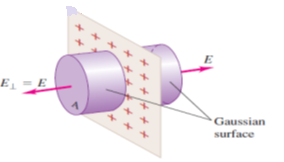$\varphi$(left) + $\varphi$(right)=\$ =EA Cos0+EA Cos0=2EA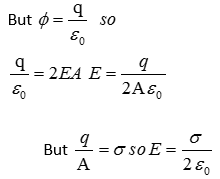Workspace
Report
Q.8
When a negatively charged conductor is connected to earth,
A. Electrons flow from the conductor to the earth
B. Protons flow from the conductor to the earth
C. No charge flow occurs.
D. Electrons flow from the earth to the conductor
Explaination / Solution:

After earthing a positively charged conductor electrons flow from earth to conductor and if a negatively charged conductor is earthed then electrons flows from conductor to earth.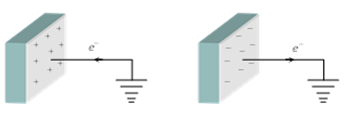Workspace
Report
Q.9
Eight dipoles of charges of magnitude e are placed inside a cube. The total electric flux coming out of the cube will be
A. A .
B. 16eϵo
C. Zero
D. eϵo
Explaination / Solution:

On all the dipoles net charge=0 so theotal electric flux coming out of the surface
Workspace
Report
Q.10
Two insulated charged copper spheres A and B have their centres separated by a distance of 50 cm and have identical sizes. A third sphere of the same size but uncharged is brought in contact with the first, then brought in contact with the second, and finally removed from both. What is the new force of repulsion between A and B?
A. 6.7×103/N6.7×103/N
B. 3.7×103/N3.7×103/N
C. 5.7×103/N
D. 4.7×103/N